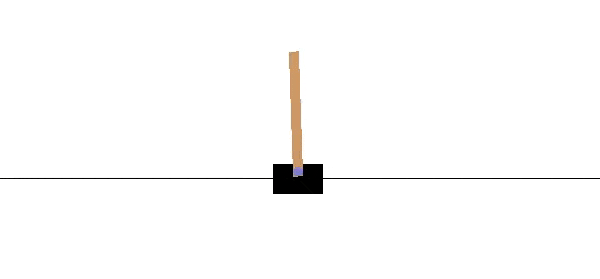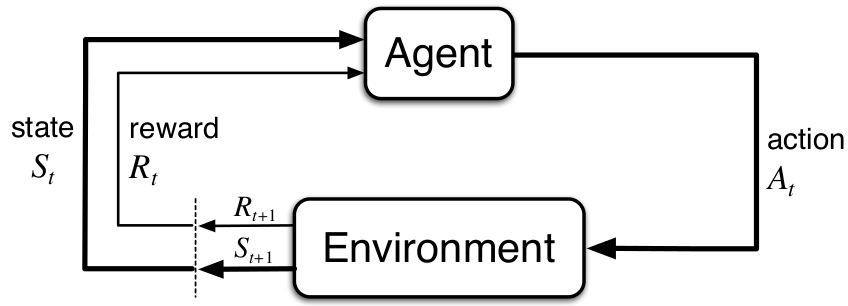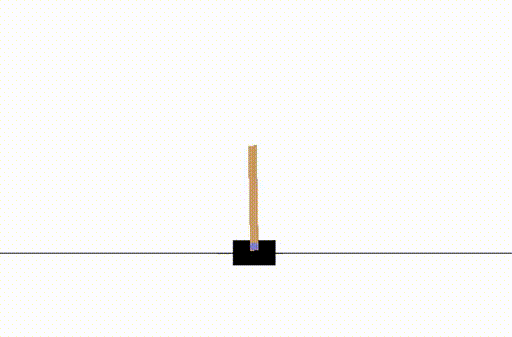# Coding period week 1

I spent the community bonding period learning more about Jderobot’s Behavior Studio which focuses on using deep learning for self driving cars. It was also great to talk to my mentors and meet other members from Behavior Studio, they are all very kind and supportive. and know more about the Jderobot community.

The first week of the coding period, I started by studying the tensorflow agents library which has a set of great tutorials, and implemented some basic examples with Deep Q-Networks (DQN), Additionally I set up a Dockerfile to ease my work.

Exploring tensorflow agents and DQN in an easier environment like cartpole, would help me translate the implementation to more complex environment like Formula 1 environment. Additionally, I set the environment where I will be working using a GPU.## Reinforcement LearningFigure the book, Reinforcement Learning: An Introduction by Andrew Barto and Richard S. Sutton

In a previous post I wrote more about it, but to sum up reinforcement learning (RL) algorithms unlike supervised learning, learns from trial and error.

As shown in the figure, an agent acts based in an observation given by the environment, this action gives the agent a new observation and a reward signal which is a way to tell the agent how good was the action taken at that particular observation.

## Q-Learning

A $Q^{*}$ represents the optimal return to get from a state $s$ and an action $a$ also denoted as $Q^{*}(s, a)$. Hence in Q-Learning we tried to get the $Q_{*}$ for each step, it can be done using a simple matrix mapping states and actions.

The bellman equation is used to approximate to the optimal value $Q^{*}$, taking the reward $r$ in the current state $s$ and the maximum $Q^{*}$ of the next state $s’$ discounted by gamma $\gamma$ .

$\begin{equation}Q^{*}(s, a) = \mathbb{E}\left[ r + \gamma \max_{a'} Q^{*}(s', a') \right]\end{equation}$

## Deep Q-Network

Deep Q-Network was developed by Deepmind in 2015, combining deep neural networks and Q-learning .

More complex environments like games or embodied robots using cameras generate observation that cannot longer be stores in a tabular setting, hence deep learning comes to the rescue.

# Implemented

## Environment

I mainly used tensorflow 2 and tensorflow agents which works with openai-gym!. An environment from gym can easily be loaded to tf-agents by using suite_gym.load then this python environment that uses numpy arrays can be converted to a tensor environment.

env = suite_gym.load('CartPole-v0')
train_env = tf_py_environment.TFPyEnvironment(env)


## Network

QNetwork class provides by tf-agents makes easy to implement the DQN, here we are creating from the specifications of the Carpole environment with a fully connected layer with 100 units.

fc_layer_params = (100,)

q_net = q_network.QNetwork(
train_env.observation_spec(),
train_env.action_spec(),
fc_layer_params=fc_layer_params)


## Agent

Just like QNetwork there is a class DqnAgent uses the q_net previously instantiated, an optimizer and the time step specifications. Later on it would be easy to try new agents that are already implemented in the library.

optimizer = tf.compat.v1.train.AdamOptimizer(learning_rate=learning_rate)
train_step_counter = tf.Variable(0)

agent = dqn_agent.DqnAgent(
train_env.time_step_spec(),
train_env.action_spec(),
q_network=q_net,
optimizer=optimizer,
td_errors_loss_fn=common.element_wise_squared_loss,
train_step_counter=train_step_counter)

agent.initialize()


## Policy

Policies are the behavior that our agent would agent, some policies are already predefined like the random_policy for exploration.

eval_policy = agent.policy
collect_policy = agent.collect_policy
random_policy = random_tf_policy.RandomTFPolicy(
train_env.time_step_spec(),
train_env.action_spec())


## Replay Buffer

The replay buffer is an important part in the DQN algorithms this is where some trajectories are stored, basically it stores tuples of observations, reward, and actions, and the next state or observation. Then the tuples are sampled during training to about correlation between consecutive states, and make more efficient use of previous experience.

Initially the collect_data function gathers n_step into the buffer using the random policy, also the function as_dataset allow us to iterate (sample) over the replay buffer.

replay_buffer = tf_uniform_replay_buffer.TFUniformReplayBuffer(
data_spec=agent.collect_data_spec,
batch_size=train_env.batch_size,
max_length=replay_buffer_max_length)

collect_data(train_env, random_policy, replay_buffer, n_steps=initial_collect_steps)

dataset = replay_buffer.as_dataset(
num_parallel_calls=3,
sample_batch_size=batch_size,
num_steps=2).prefetch(3)

iterator = iter(dataset)


## Training

After around ~5min the agent completes 20000 steps and its policy achieves the maximum score 200.

# (Optional) Optimize by wrapping some of the code in a graph using TF function.
agent.train = common.function(agent.train)

# Evaluate the agent's policy once before training.
avg_return = compute_avg_return(eval_env, agent.policy, num_eval_episodes)
returns = [avg_return]

for _ in range(num_iterations):

# Collect a few steps using collect_policy and save to the replay buffer.
for _ in range(collect_steps_per_iteration):
collect_step(train_env, agent.collect_policy, replay_buffer)

# Sample a batch of data from the buffer and update the agent's network.
experience, unused_info = next(iterator)
train_loss = agent.train(experience).loss

step = agent.train_step_counter.numpy()


Since this environment has only to possible actions (left and right), and its observation space are four floats, it is fairly easy to achieve good results in such amount of time.## Week Highlights

• Studied thoughtfully the tf-agents library which is going to accelerate the development of Deep Reinforcement Algorithms in JdeRobot environments.
• Implemented a DQN example in the CartPole environment testing the working environment I set up using GPUs in a container along with JdeRobot Libraries.
• Started the DQN implementation of a environment (BreakOut) which uses images since the Jderobot’s Formula 1 environment uses a camera for perception.
• Modularized the implementations and added documentation to ease replication.
• Met with my mentors and the Behavior Studio Team, I understood better the project and how work is going to fit the Behavior Studio tools.
• Even using docker I had a little bit of trouble to met tensorflow-gpu dependencies, and besides using a GPU training times for DRL last hours so it takes some time to debug.

 Deepmind, DQN (Deep Q-Network) algorithm, 2015.

 Sergio Guadarrama et at, TF-Agents A library for Reinforcement Learning in TensorFlow, 2018.

 Richard S. Sutton and Andrew G. Barto, Reinforcement Learning: An Introduction, 2018